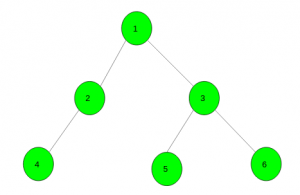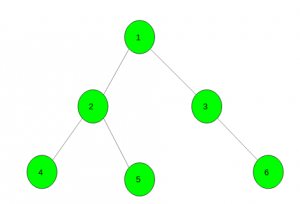Open in App
Not now

# Minimum steps to color the tree with given colors

• Difficulty Level : Hard
• Last Updated : 03 Jun, 2021

Given a tree with N nodes which initially have no color and an array color[] of size N which represent the color of each node after the coloring process takes place. The task is to color the tree into the given colors using the smallest possible number of steps. On each step, one can choose a vertex v and a color x, and then color all vertices in the sub-tree of v (including v itself) with color x. Note that root is vertex number 1.
Examples:

Input: color[] = { 1, 1, 2, 1, 3, 1}Output:
Color the sub-tree rooted at node 1 with color 1.
Then all the vertices have colors 1.
Now, color the sub-tree rooted at 3 with color 2.
Finally, color the sub-trees rooted at 5 and 6 with colors 3 and 1 respectively.
Input: color[] = { 1, 2, 3, 2, 2, 3}Output:

Approach: Call a DFS function at vertex 1 and initially keep answer as zero. Increment the answer whenever there is a difference in colors of child and parent nodes.
See the below code for better understanding.
Below is the implementation of the above approach:

## C++

 `// C++ implementation of the approach` `#include ` `using` `namespace` `std;`   `// To store the required answer` `int` `ans = 0;`   `// To store the graph` `vector<``int``> gr;`   `// Function to add edges` `void` `Add_Edge(``int` `u, ``int` `v)` `{` `    ``gr[u].push_back(v);` `    ``gr[v].push_back(u);` `}`   `// Dfs function` `void` `dfs(``int` `child, ``int` `par, ``int` `color[])` `{`   `    ``// When there is difference in colors` `    ``if` `(color[child] != color[par])` `        ``ans++;`   `    ``// For all it's child nodes` `    ``for` `(``auto` `it : gr[child]) {` `        ``if` `(it == par)` `            ``continue``;` `        ``dfs(it, child, color);` `    ``}` `}`   `// Driver code` `int` `main()` `{`   `    ``// Here zero is for parent of node 1` `    ``int` `color[] = { 0, 1, 2, 3, 2, 2, 3 };`   `    ``// Adding edges in the graph` `    ``Add_Edge(1, 2);` `    ``Add_Edge(1, 3);` `    ``Add_Edge(2, 4);` `    ``Add_Edge(2, 5);` `    ``Add_Edge(3, 6);`   `    ``// Dfs call` `    ``dfs(1, 0, color);`   `    ``// Required answer` `    ``cout << ans;`   `    ``return` `0;` `}`

## Java

 `// Java implementation of the approach ` `import` `java.util.*;`   `class` `GFG` `{`   `// To store the required answer ` `static` `int` `ans = ``0``; `   `// To store the graph ` `static` `Vector> gr = ``new` `Vector>(); `   `// Function to add edges ` `static` `void` `Add_Edge(``int` `u, ``int` `v) ` `{ ` `    ``gr.get(u).add(v); ` `    ``gr.get(v).add(u); ` `} `   `// Dfs function ` `static` `void` `dfs(``int` `child, ``int` `par, ``int` `color[]) ` `{ `   `    ``// When there is difference in colors ` `    ``if` `(color[child] != color[par]) ` `        ``ans++; `   `    ``// For all it's child nodes ` `    ``for` `(``int` `i = ``0``; i < gr.get(child).size(); i++)` `    ``{ ` `        ``if` `(gr.get(child).get(i) == par) ` `            ``continue``; ` `        ``dfs(gr.get(child).get(i), child, color); ` `    ``} ` `} `   `// Driver code ` `public` `static` `void` `main(String args[])` `{ ` `    ``for``(``int` `i = ``0``; i <= ``10``; i++)` `    ``gr.add(``new` `Vector());`   `    ``// Here zero is for parent of node 1 ` `    ``int` `color[] = { ``0``, ``1``, ``2``, ``3``, ``2``, ``2``, ``3` `}; `   `    ``// Adding edges in the graph ` `    ``Add_Edge(``1``, ``2``); ` `    ``Add_Edge(``1``, ``3``); ` `    ``Add_Edge(``2``, ``4``); ` `    ``Add_Edge(``2``, ``5``); ` `    ``Add_Edge(``3``, ``6``); `   `    ``// Dfs call ` `    ``dfs(``1``, ``0``, color); `   `    ``// Required answer ` `    ``System.out.println( ans); ` `} ` `} `   `// This code is contributed by Arnab Kundu`

## Python3

 `# Python3 implementation of the approach`   `# To store the required answer` `ans ``=` `0`   `# To store the graph` `gr ``=` `[[] ``for` `i ``in` `range``(``100005``)]`   `# Function to add edges` `def` `Add_Edge(u, v):` `    ``gr[u].append(v)` `    ``gr[v].append(u)`   `# Dfs function` `def` `dfs(child, par, color):` `    ``global` `ans`   `    ``# When there is difference in colors` `    ``if` `(color[child] !``=` `color[par]):` `        ``ans ``+``=` `1`   `    ``# For all it's child nodes` `    ``for` `it ``in` `gr[child]:` `        ``if` `(it ``=``=` `par):` `            ``continue` `        ``dfs(it, child, color)` `    `  `# Driver code`   `# Here zero is for parent of node 1` `color ``=` `[``0``, ``1``, ``2``, ``3``, ``2``, ``2``, ``3``]`   `# Adding edges in the graph` `Add_Edge(``1``, ``2``)` `Add_Edge(``1``, ``3``)` `Add_Edge(``2``, ``4``)` `Add_Edge(``2``, ``5``)` `Add_Edge(``3``, ``6``)`   `# Dfs call` `dfs(``1``, ``0``, color)`   `# Required answer` `print``(ans)`   `# This code is contributed ` `# by mohit kumar`

## C#

 `// C# implementation of the approach ` `using` `System;` `using` `System.Collections.Generic;`   `class` `GFG` `{`   `    ``// To store the required answer ` `    ``static` `int` `ans = 0; ` `    `  `    ``// To store the graph ` `    ``static` `List> gr = ``new` `List>(); ` `    `  `    ``// Function to add edges ` `    ``static` `void` `Add_Edge(``int` `u, ``int` `v) ` `    ``{ ` `        ``gr[u].Add(v); ` `        ``gr[v].Add(u); ` `    ``} ` `    `  `    ``// Dfs function ` `    ``static` `void` `dfs(``int` `child, ``int` `par, ``int` `[]color) ` `    ``{ ` `    `  `        ``// When there is difference in colors ` `        ``if` `(color[child] != color[par]) ` `            ``ans++; ` `    `  `        ``// For all it's child nodes ` `        ``for` `(``int` `i = 0; i < gr[child].Count; i++)` `        ``{ ` `            ``if` `(gr[child][i] == par) ` `                ``continue``; ` `            ``dfs(gr[child][i], child, color); ` `        ``} ` `    ``} `   `    ``// Driver code ` `    ``public` `static` `void` `Main(String []args)` `    ``{ ` `        ``for``(``int` `i = 0; i <= 10; i++)` `        ``gr.Add(``new` `List<``int``>());` `    `  `        ``// Here zero is for parent of node 1 ` `        ``int` `[]color = { 0, 1, 2, 3, 2, 2, 3 }; ` `    `  `        ``// Adding edges in the graph ` `        ``Add_Edge(1, 2); ` `        ``Add_Edge(1, 3); ` `        ``Add_Edge(2, 4); ` `        ``Add_Edge(2, 5); ` `        ``Add_Edge(3, 6); ` `    `  `        ``// Dfs call ` `        ``dfs(1, 0, color); ` `    `  `        ``// Required answer ` `        ``Console.WriteLine( ans); ` `    ``} ` `}`   `// This code has been contributed by 29AjayKumar`

## Javascript

 ``

Output:

`3`

My Personal Notes arrow_drop_up
Related Articles